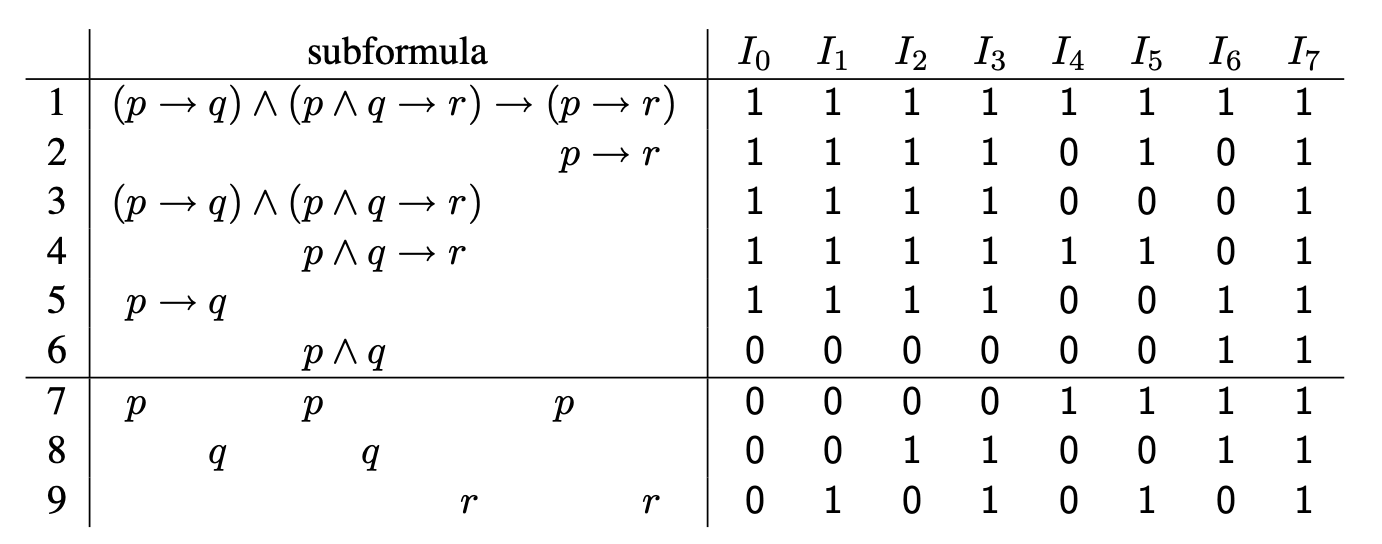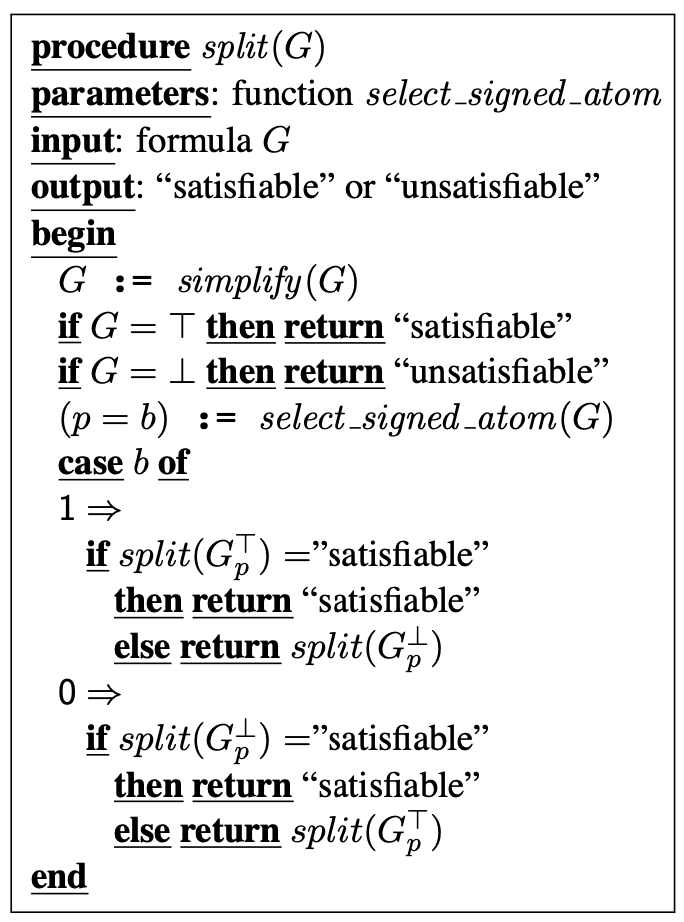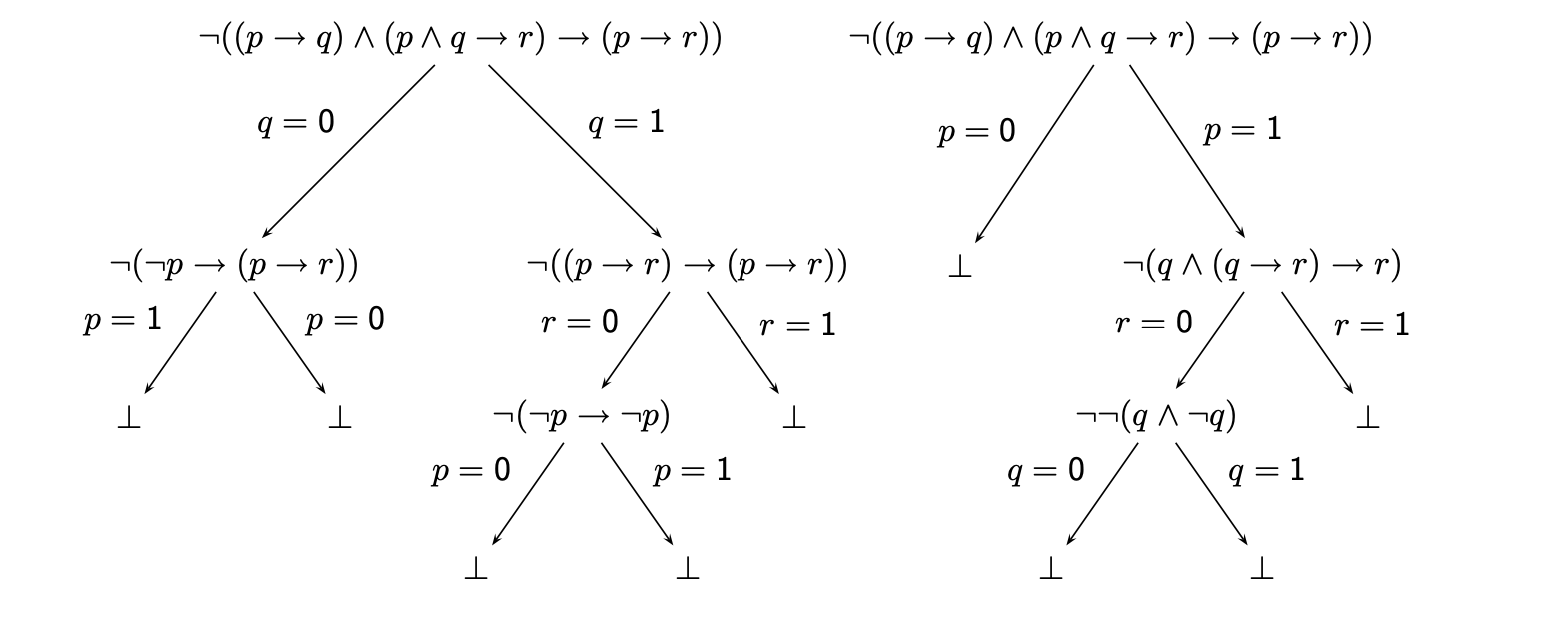# 逻辑学探幽 Part4

## 没有逻辑 只有heip

Posted by R1NG on October 1, 2021 Viewed Times

# 可满足性检验

## 4.1 真值表## 4.2 分割法

1. 若 $T \vDash \neg p$, 则 $A$ 在解释 $I$ 中和 $A_{p}^{\perp}$ 等价.

2. 若 $T \vDash p$, 则 $A$ 在解释 $I$ 中和 $A_{p}^{\top}$ 等价.

$I'(q) \overset{\text{def}}{=} \begin{cases} I(q) ~~~ \text{if} ~ p \neq q \\ 1~~~~~~~~\text{if} ~ p=q \end{cases}$

1. 对任意公式 $A$ 和解释 $I$, $A=1$ 和 $A=0$ 中恰有一个为真.

2. 公式 $A$ 为可满足的, 当且仅当有符号公式 $A=1$ 为可满足的.1. 若给定的公式 $G$ 为可满足的, 则分割算法会终止并返回结果 satisfiable.
2. 若给定的公式 $G$ 为不可满足的, 则分割算法会终止并返回结果 unsatisfiable.

## 4.3 子公式的极性

$0 \leqslant 0, ~ 0 \leqslant 1, ~ 1 \leqslant 0,~ 1 !\leqslant 0.$

$f(v_1, \cdots, v_k, \cdots, v_n) \leqslant f(v_1, \cdots, v'_k, \cdots, v_n)$

$n=0$ 时序列为空, 我们称其为 空位置, 记为 $\epsilon$. 极性 为一个可取 $-1, 0, 1$ 的值.

1. 给定公式中的位置
2. 位于公式 $A$ 的位置 $\pi$ 处的子公式 $A\vert_{\pi}$
3. 位于公式 $A$ 的位置 $\pi$ 处的子公式 $A\vert_{\pi}$ 的极性 $\text{pol}(A, \pi)$

i. 对任何公式 $A$, $\epsilon$ 为 $A$ 中的一个位置, 且有

$A\vert_{\epsilon} \overset{\text{def}}{=} A, ~ \text{pol}(A, \pi) = 1.$

ii. 令 $A\vert_{\pi} = B$:

• 若子公式 $B$ 形如 $B_1, \wedge \cdots \wedge B_n$ 或 $B_1, \vee \cdots \vee B_n$, 则对 $\forall ~ i \in [n]$: 位置 $\pi.i$ 位于 $A$ 中, $A \vert_{\pi.i} \overset{\text{def}}{=} B_i$, 且有 $\text{pol}(A, \pi.i) \overset{\text{def}}{=} \text{pol}(A, \pi)$.

• 若子公式 $B$ 形如 $\neg B_1$, 则 $\pi.1$ 为 $A$ 中的一个位置, $A\vert_{\pi.1} \overset{\text{def}}{=} B_1$, 且有 $\text{pol}(A, \pi.1) \overset{\text{def}}{=} -\text{pol}(A, \pi)$.

• 若子公式 $B$ 形如 $B_1 \rightarrow B_2$, 则 $\pi.1, \pi.2$ 为 $A$ 中的一个位置, $A\vert_{\pi.1} \overset{\text{def}}{=} B_1$, $A\vert_{\pi.2} \overset{\text{def}}{=} B_2$, 且有 $\text{pol}(A, \pi.1) \overset{\text{def}}{=} -\text{pol}(A, \pi)$, $\text{pol}(A, \pi.2) \overset{\text{def}}{=} \text{pol}(A, \pi)$.

• 若子公式 $B$ 形如 $B_1 \leftrightarrow B_2$, 则 $\pi.1, \pi.2$ 为 $A$ 中的一个位置, $A\vert_{\pi.1} \overset{\text{def}}{=} B_1$, $A\vert_{\pi.2} \overset{\text{def}}{=} B_2$, 且有 $\text{pol}(A, \pi.1) \overset{\text{def}}{=} \text{pol}(A, \pi.2) =0$.

1. 若子公式 $B$ 在逻辑运算符 $\leftrightarrow$ 的任一个域 (也就是左边或右边) 出现, 则位置$\pi$ 的极性为 $0$.

2. 若子公式 $B$ 不在逻辑运算符 $\leftrightarrow$ 的任一个域 (也就是左边或右边) 出现, 则位置$\pi$ 的极性为 $1$ (相应地, $-1$). 当且仅当 $B$ 在逻辑运算符 $\neg$ 的域中出现, 或在运算符 $\rightarrow$ 的左侧域中出现了偶数次 (相应地, 奇数次).

$I(A) \leqslant I(B)$

$I \vDash A \rightarrow B.$

$\text{pol}(A, \pi) = 1,$

$I \vDash A[B]_{\pi} \rightarrow A[B']_{\pi}.$

$\text{pol}(A, \pi) = -1,$

$I \vDash A[B']_{\pi} \rightarrow A[B]_{\pi}.$

$I'(q) \overset{\text{def}}{=} \begin{cases} 1, ~~~~~~~\text{if} ~ p=q~ \text{and} ~p~ \text{occurrs in}~A~\text{only positively.}\\ 0, ~~~~~~~ \text{if} ~ p=q~ \text{and} ~p~ \text{occurrs in}~A~\text{only negatively.} \\ I(q)~~~~ \text{if}~p \neq q.\end{cases}$

$A^{\top}_{p} ~~ (A^{\perp}_{p})$

$A \rightarrow A^{\top}_{p}$

$I' \vDash p \leftrightarrow \top.$